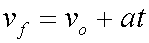# Introduction to the Velocity and Acceleration Equation

This equation relates final velocity, original velocity, constant acceleration, and time:The final velocity equals the original velocity plus the acceleration multiplied by the time.

Here is a sample problem and its solution demonstrating the use of this equation:

An object is moving with a velocity of 4.0 m/s when it begins to accelerate. It accelerates at 3.0 m/s/s, (3.0 m/s2), for a time period of 5.0 s. What is the object's final velocity after the acceleration?

 vf = vo + at Begin here. vf = 4.0 m/s + (3.0 m/s/s)(5.0 s) Plug in values with units. vf = 4 + 15 Do some intermediate mathematics. vf = 19 m/s Come up with a final result.

So, this object is moving with a velocity of 19 m/s after the acceleration.

More:

You can use the definition of acceleration to understand the origin of this equation .

Using algebra this equation can be rearranged and solved for any of the variables present in it.

There are equivalent alternate symbols for the quantities in this equation.

Custom Search